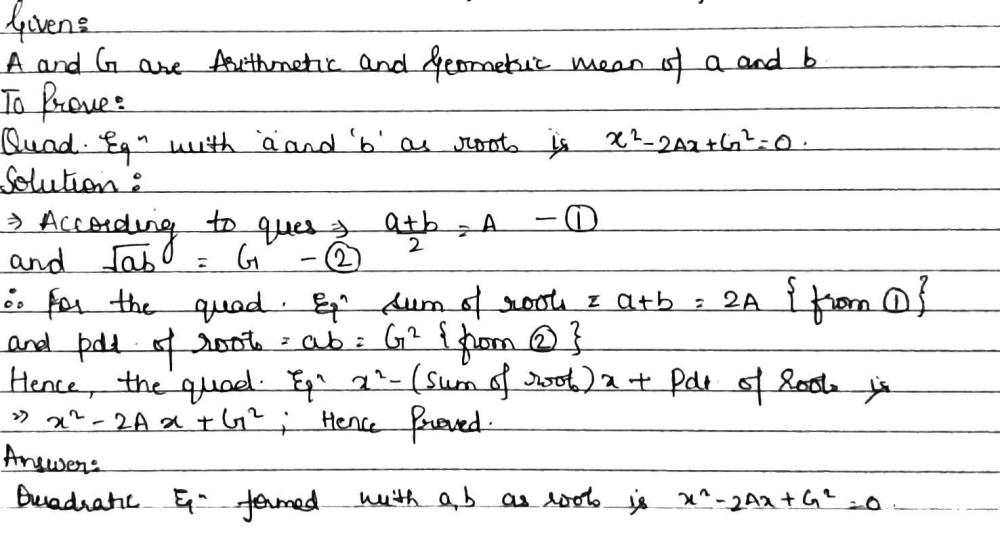Home/Class 11/Maths/

## QuestionMathsClass 11

If A and G are respectively arithmetic and geometric mean between positive no. a and b; then the quadratic equation having a, b as its roots is
$${x}^{2}-2Ax+ {G}^{2}=0$$